• 相干信号
千次阅读
2020-06-29 19:23:59

相干信号：

两束满足相干条件的信号称为相干信号，
相干条件（Coherent Condition）：
这两束信号在相遇区域：①振动方向相同；
②振动频率相同；
③相位相同或相位差保持恒定

相干积分：

于输入进导航接收机的数字中频信号，首先与NCO输出的正弦信号和余弦信号分别混频相乘。得到对应于同相支路和正交支路的两个积分值。经过相关器后进行积分-清除运算。积分-清除器通过积分低通滤波器消除信号中的高频信号成分和噪声，以提高载噪比。 

在同相支路和正交支路上的积分-清除电路的工作过程大致如下：积分器对输入信号经过一定时间的积分后，分别输出两条支路的积分结果，然后清除器清除积分器中的各个寄存单元，接着再进行下一时段的积分，如此重复不断。

非相干积分：

非相干积分把本地码与卫星信号的相关程度累 加，这个过程即本地码与卫星信号的逐步同步，当同步达到要求后， 即累加值达到了阈值。在捕获的过程中，通常是对相关的处理结果进 行非相干的处理，非相干积分是使用了相关积分的结果，以达到提高 信噪比的作用。

博主的闲聊群

QQ:701359719更多相关内容
• 实现相干信号源的方位估计，提高相干信号源的估计精度
• 首先分析了基于非相干信号子空间(ISM)的宽带源DOA估计方法，并将基于数据阵共轭重构的修正MUSIC算法应用于ISM方法中，提高了分辨能力而且可以分辨相干信源。接下来讨论了相干信号子空间(CSM)方法的宽带相干源DOA估计...
• 基于非相干信号字空间宽带源DOA估计方法，代码可运行
• 相干信号源music算法当接收的信号源是部分相干或者全部相干的时候，信号源协方差矩阵会出现亏秩的情况，进而导致阵列接收数据协方差矩阵出现缩维的情况，所以基于数据协方差矩阵特征分解的子空间类算法性能会变得很...
• 到达方向（DOA）估计是阵列... 最后，通过利用空间差分矩阵来估计剩余的相干信号。 与以前的工作相比，该方法可以提高DOA估计的准确性，并且可以增加可检测信号的最大数目。 理论分析和仿真结果证实了该方法的有效性。
• 该程序用于宽带非相干信号doa估计，即ISM算法，经实验绝对可用
• 为了解决低信噪比下相干信号波达方向(DOA)估计精度和分辨率不高的问题，提出了低信噪比下相干信号DOA估计算法，此方法主要利用了空间平滑算法和ES-MUSIC算法的优点。相干信号源进行解相干通过空间平滑技术实现，接着...
• 利用MATLAB实现了相干信号源的DOA估计算法对比，包括Music，空间平滑Music，双向空间平滑Music，传统LSESPRIT，TEOPLITZ-ESPRIT算法对比
• 利用MATLAB实现了相干信号源的MUSIC算法，给出最终估计的空间谱，对了解相干信号源的MUSIC算法以及DOA估计有一定的帮助
• 为解决分子通信中由分子扩散带来的码间干扰（ISI）和背景噪声这2个严峻的挑战，采用4种能抵抗ISI的信号特征表示接收信号的瞬态特性，提出了可靠的不依赖于信道冲激响应（CIR）的非相干分子信号检测算法，并设计了...码间干扰 特征提取
• 虚拟MIMO系统中不相关和相干信号的高效能源DOA估计算法。研究论文
• 1、实现效果：见链接：...2、调频信号FM相干解调与非相干解调原理matlab实现，并采用matlab GUI界面实现，包括了AM&FM&DPSK&FSK 的调制解调。 3、适用于计算机，电子信息工程等专业的大学生毕业设计。
• 在信号的传输过程中，由于信号反射和折射，导致多径传输产生相干信号。此时信号协方差矩 阵出现秩缺，导致传统的超分辨波达方向估计（Ｄｉｒｅｃｔｉｏｎ　ｏｆ　ａｒｒｉｖａｌ，ＤＯＡ）算法失效。针对相干信号的 ...
• 前后项空间平滑算法，用于相干信号的解相干，即相干信号的波达方向估计
• 基于空间平滑MUSIC算法的相干信号DOA估计(1)基于空间平滑MUSIC算法的相干信号DOA估计(1)空间平滑MUSIC算法(1)在上一篇博客中有提到，当多个入射信号相干时，传统MUSIC算法的效果就会不理想。具体原因是多个入射信号...

基于空间平滑MUSIC算法的相干信号DOA估计(1)

基于空间平滑MUSIC算法的相干信号DOA估计(1)

空间平滑MUSIC算法(1)

在上一篇博客中有提到，当多个入射信号相干时，传统MUSIC算法的效果就会不理想。具体原因是多个入射信号相干时，有部分能量就会散发到噪声子空间，使得MUSIC算法不能对其进行有效估计。

针对这种情况，解相干方法被提出。本文主要讲解通过降维处理解相干，之所以叫降维处理是因为这种方法将原阵列拆分成很多个子阵列，通过子阵列的协方差矩阵重构接收数据协方差矩阵，阵列的自由度DOF会随之降低，即可分辨的相干信号数降低。

先看看传统MUSIC算法对相干信号进行DOA估计的效果。

MATLAB代码

% Code For Music Algorithm

% The Signals Are All Coherent

% Author:痒羊羊

% Date：2020/10/29

clc; clear all; close all;

%% -------------------------initialization-------------------------

f = 500; % frequency

c = 1500; % speed sound

lambda = c/f; % wavelength

d = lambda/2; % array element spacing

M = 20; % number of array elements

N = 100; % number of snapshot

K = 6; % number of sources

coef = [1; exp(1i*pi/6);...

exp(1i*pi/3); exp(1i*pi/2);...

exp(2i*pi/3); exp(1i*2*pi)]; % coherence coefficient, K*1

doa_phi = [-30, 0, 20, 40, 60, 75]; % direction of arrivals

%% generate signal

dd = (0:M-1)'*d; % distance between array elements and reference element

A = exp(-1i*2*pi*dd*sind(doa_phi)/lambda); % manifold array, M*K

S = sqrt(2)\(randn(1,N)+1i*randn(1,N)); % vector of random signal, 1*N

X = A*(coef*S); % received data without noise, M*N

X = awgn(X,10,'measured'); % received data with SNR 10dB

%% calculate the covariance matrix of received data and do eigenvalue decomposition

Rxx = X*X'/N; % covariance matrix

[U,V] = eig(Rxx); % eigenvalue decomposition

V = diag(V); % vectorize eigenvalue matrix

[V,idx] = sort(V,'descend'); % sort the eigenvalues in descending order

U = U(:,idx); % reset the eigenvector

P = sum(V); % power of received data

P_cum = cumsum(V); % cumsum of V

%% define the noise space

J = find(P_cum/P>=0.95); % or the coefficient is 0.9

J = J(1); % number of principal component

Un = U(:,J+1:end);

%% music for doa; seek the peek

theta = -90:0.1:90; % steer theta

doa_a = exp(-1i*2*pi*dd*sind(theta)/lambda); % manifold array for seeking peak

music = abs(diag(1./(doa_a'*(Un*Un')*doa_a))); % the result of each theta

music = 10*log10(music/max(music)); % normalize the result and convert it to dB

%% plot

figure;

plot(theta, music, 'linewidth', 2);

title('Music Algorithm For Doa', 'fontsize', 16);

xlabel('Theta(°)', 'fontsize', 16);

ylabel('Spatial Spectrum(dB)', 'fontsize', 16);

grid on;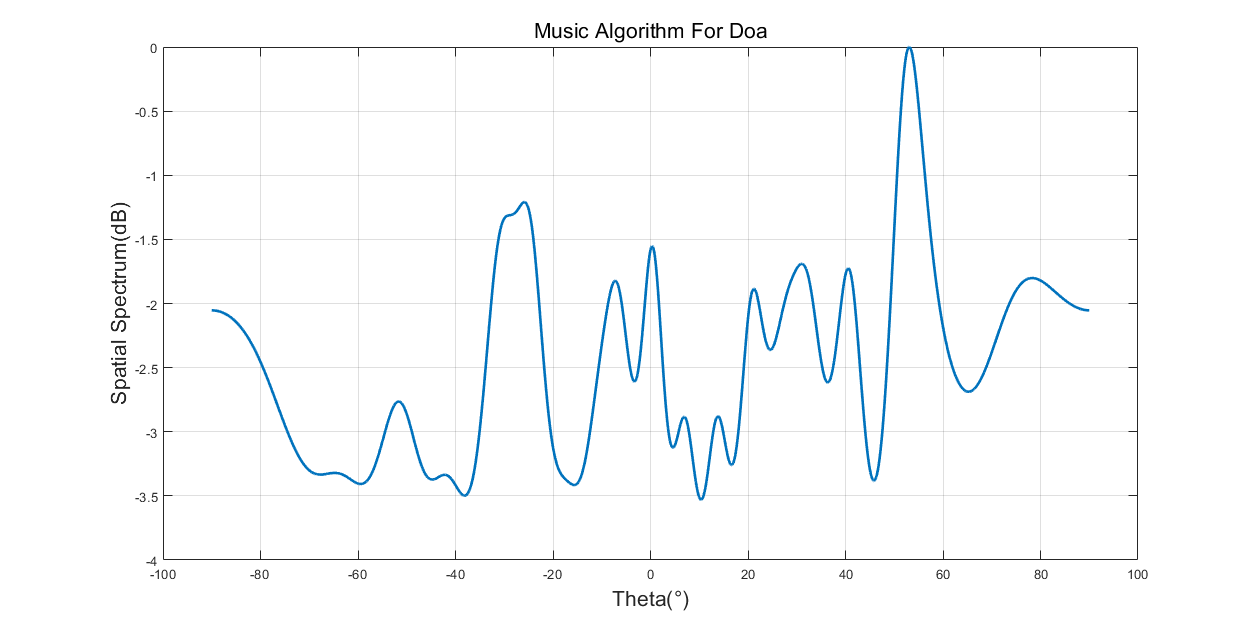可以看到，对于相干信号，传统MUSIC算法DOA估计效果很差。

降维处理解相干方法主要包括空间平滑类处理算法，而空间平滑类处理算法可以分成前向空间平滑算法(FSS)、后向平滑算法(BSS)、前后向平滑算法(FBSS)，正如上面所说，这些算法的估计效果很好，但是损失了阵列孔径，导致可分辨的相干信号数降低。

2.1 线性阵列信号模型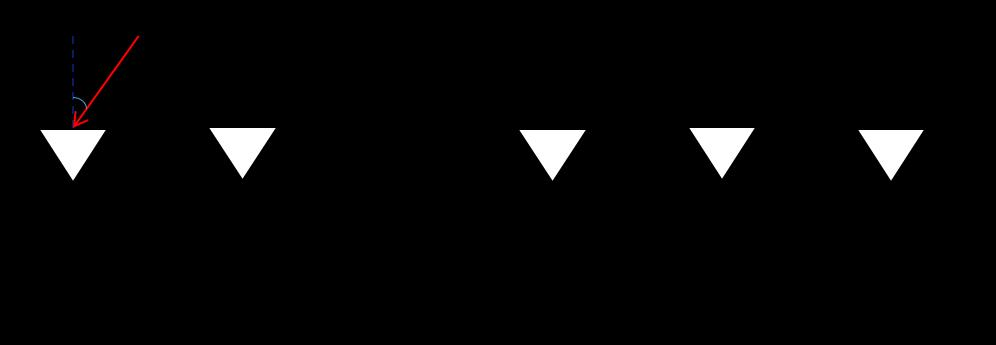关于这个线性阵列中符号的具体说明可以参考上一篇博文。子空间方法——MUSIC算法

2.2 前向空间平滑算法

前向空间平滑算法将阵列分成多个互相重叠的子阵列，然后对子阵接收数据的协方差矩阵作平均。当子阵阵元数目大于等于相干信号数时，可以有效解相干。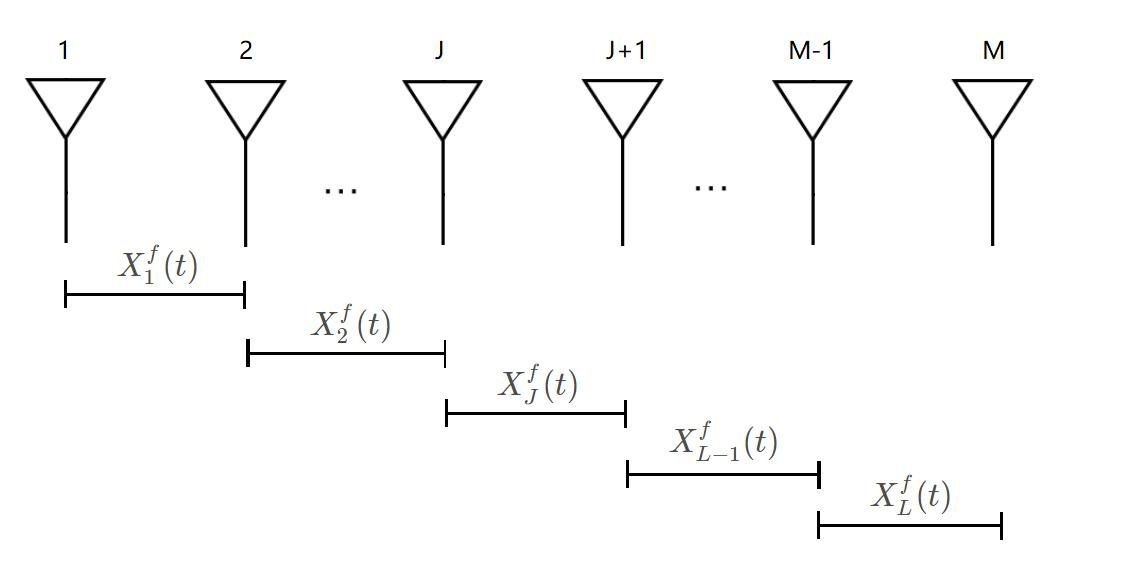如上图所示，我们把M元阵列均匀分成L个子阵列，每个子阵列有N=M-L+1个阵元。以最左边的子阵列为参考阵列，定义第J个子阵的接收数据为：

X

J

f

=

[

x

J

,

x

J

+

1

,

?

?

,

x

J

+

N

?

1

]

X_{J}^{f}=\left[x_{J}, x_{J+1}, \cdots, x_{J+N-1}\right]

XJf?=[xJ?,xJ+1?,?,xJ+N?1?]那么第J个子阵接收数据的协方差矩阵(也称作空间平滑矩阵)可以表示为

R

N

j

j

=

A

1

D

j

?

1

R

s

(

D

j

?

1

)

H

A

1

H

+

σ

2

I

N

j

=

1

,

2

,

?

?

,

L

R_{N}^{j j}=A_{1} D^{j-1} R_{s}\left(D^{j-1}\right)^{H} A_{1}^{H}+\sigma^{2} I_{N} \quad j=1,2, \cdots, L

RNjj?=A1?Dj?1Rs?(Dj?1)HA1H?+σ2IN?j=1,2,?,L其中，

D

=

diag

?

[

e

?

j

?

2

π

d

λ

sin

?

θ

1

,

e

?

j

2

π

d

λ

sin

?

θ

2

,

?

e

?

j

2

π

d

λ

sin

?

θ

R

]

D=\operatorname{diag}\left[\mathrm{e}^{-j\frac{-2 \pi d}{\lambda} \sin \theta_{1}}, \mathrm{e}^{-j\frac{2 \pi d}{\lambda} \sin \theta_{2}}, \cdots \mathrm{e}^{-j\frac{2 \pi d}{\lambda} \sin \theta_{R}}\right]

D=diag[e?jλ?2πd?sinθ1?,e?jλ2πd?sinθ2?,?e?jλ2πd?sinθR?]，A1为第一个子阵即参考阵列的流型矩阵。

因此，前向空间平滑后的协方差矩阵可以由各个子阵的协方差矩阵求平均得到。

R

~

f

=

1

L

j

=

1

L

R

N

j

j

\tilde{R}^{f}=\frac{1}{L} \sum_{j=1}^{L} R_{N}^{j j}

R~f=L1?j=1∑L?RNjj?利用前向空间平滑协方差矩阵和MUSIC算法就可以分辨出多个相干信号的方位。可以证明，该方法最大可以检测M/2个相干的信号。

MATLAB代码

% Code For Music Algorithm

% The Signals Are All Coherent

% Author:痒羊羊

% Date：2020/10/29

clc; clear all; close all;

%% -------------------------initialization-------------------------

f = 500; % frequency

c = 1500; % speed sound

lambda = c/f; % wavelength

d = lambda/2; % array element spacing

M = 20; % number of array elements

N = 100; % number of snapshot

K = 6; % number of sources

L = 10; % number of subarray

L_N = M-L+1; % number of array elements in each subarray

coef = [1; exp(1i*pi/6);...

exp(1i*pi/3); exp(1i*pi/2);...

exp(2i*pi/3); exp(1i*2*pi)]; % coherence coefficient, K*1

doa_phi = [-30, 0, 20, 40, 60, 75]; % direction of arrivals

%% generate signal

dd = (0:M-1)'*d; % distance between array elements and reference element

A = exp(-1i*2*pi*dd*sind(doa_phi)/lambda); % manifold array, M*K

S = sqrt(2)\(randn(1,N)+1i*randn(1,N)); % vector of random signal, 1*N

X = A*(coef*S); % received data without noise, M*N

X = awgn(X,10,'measured'); % received data with SNR 10dB

%% reconstruct convariance matrix

%% calculate the covariance matrix of received data and do eigenvalue decomposition

Rxx = X*X'/N; % origin covariance matrix

Rf = zeros(L_N, L_N); % reconstructed covariance matrix

for i = 1:L

Rf = Rf+Rxx(i:i+L_N-1,i:i+L_N-1);

end

Rf = Rf/L;

[U,V] = eig(Rf); % eigenvalue decomposition

V = diag(V); % vectorize eigenvalue matrix

[V,idx] = sort(V,'descend'); % sort the eigenvalues in descending order

U = U(:,idx); % reset the eigenvector

P = sum(V); % power of received data

P_cum = cumsum(V); % cumsum of V

%% define the noise space

J = find(P_cum/P>=0.95); % or the coefficient is 0.9

J = J(1); % number of principal component

Un = U(:,J+1:end);

%% music for doa; seek the peek

dd1 = (0:L_N-1)'*d;

theta = -90:0.1:90; % steer theta

doa_a = exp(-1i*2*pi*dd1*sind(theta)/lambda); % manifold array for seeking peak

music = abs(diag(1./(doa_a'*(Un*Un')*doa_a))); % the result of each theta

music = 10*log10(music/max(music)); % normalize the result and convert it to dB

%% plot

figure;

plot(theta, music, 'linewidth', 2);

title('Music Algorithm For Doa', 'fontsize', 16);

xlabel('Theta(°)', 'fontsize', 16);

ylabel('Spatial Spectrum(dB)', 'fontsize', 16);

grid on;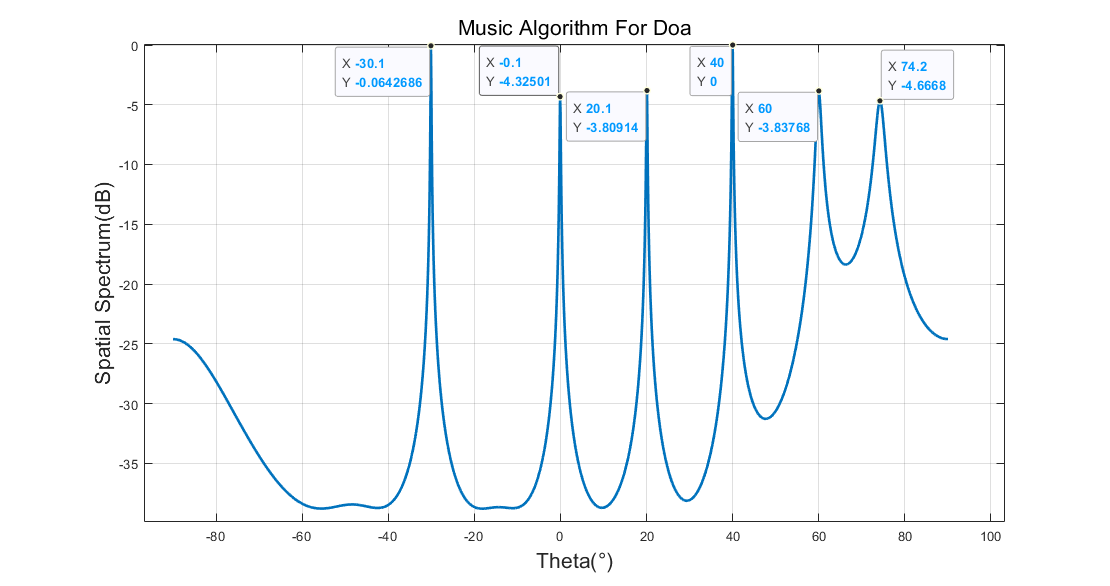可以看到，在6个入射信号均相干的情况下，基于前向平滑的MUSIC算法能较好地对其进行DOA估计，但仍存在估计精度的问题，比如真实入射角度为75°的信号的方位被估计成了74.2°。

后向空间平滑MUSIC算法和前/后向空间平滑MUSIC算法在下一篇博客中讲解。

参考论文：《基于空间平滑MUSIC算法的相干信号DOA估计，陈文锋，吴桂清》

代码Code均为原创。

欢迎转载，表明出处。

基于空间平滑MUSIC算法的相干信号DOA估计(1)相关教程

开源SQL审核查询平台Archery-基于docker安装

开源SQL审核查询平台Archery-基于docker安装 开源SQL审核查询平台Archery-基于docker安装 一、Archery产品介绍 在技术团队内部进行有效的 SQL 管理并不容易，如何进行数据库的统一管理，和线上 SQL 操作的统一审核，变得尤为重要。Archery，这个开源的 SQL 审

基于深度学习unet模型去除水印

基于深度学习unet模型去除水印 unet模型如下 def res_block(x, nb_filters, strides): res_path = BatchNormalization()(x) res_path = Activation(activation='relu')(res_path) res_path = Conv2D(filters=nb_filters, kernel_size=(3, 3), padding='sam

基于MATLAB下巴特沃斯IIR数字滤波器的实现

基于MATLAB下巴特沃斯IIR数字滤波器的实现 这里写目录标题 实验目的及要求 实验步骤 实验结果及分析 总结 实验目的及要求 1.学生自己运用MATLAB设计IIR数字低通滤波器。方法不限； 2.实现信号的滤波 参考巴特沃斯滤波器的实验原理《数字信号处理》第五章巴特

Keras深度学习应用1——基于卷积神经网络(CNN)的人脸识别(下)

Keras深度学习应用1——基于卷积神经网络(CNN)的人脸识别(下) 基于CNN的人脸识别(下) 代码下载 三、人脸识别数据集 3.1 数据集划分 3.2 数据增强 四、搭建CNN网络模型 4.1 搭建CNN模型 4.2 模型训练 4.3 加载需要识别的人脸 Keras深度学习应用1——基于卷

C++实现桶排序(基于链表)

C++实现桶排序(基于链表) 桶排序是时间复杂度为线性的排序方法,它的实现主要基于指针数组构成的链表,算法把输入数组的元素按照值的大小等分为 个区间第 个区间的元素范围是 到 所以如果我们要将值为 的元素放入桶中首先要确定桶的序号,根据上面的描述,桶的序

基于正态分布指定范围内的随机数生成算法

基于正态分布指定范围内的随机数生成算法 1、需求背景 生成一个范围(n到m，例如100到150)的随机数，但不是纯粹随机(均匀分布)的，想要的结果是基于正态分布。另外想要数字“聚集”在125左右。 生成符合正太分布的随机数不难，但是如何达到我所要求的范围？

【尚筹网IDEA版】01-基本环境空间搭建

[基于STL的演讲比赛管理系统]c++面向对象小项目

[基于STL的演讲比赛管理系统]c++面向对象小项目 头文件： SpeechManager.h #pragma once#includeiostreamusing namespace std;#includevector#includemapclass Speaker{public:string m_Name;double m_Score;};class SpeechManager{public:SpeechManager()

展开全文• 相干信号源数学模型 对两个平稳信号si(t)s_i(t)si​(t)和sk(t)s_k(t)sk​(t)，定义相关系数 信号的相关性定义如下 相干信号源间只差一个复常数，假设有nnn个相干信号源 s0(t)s_0(t)s0​(t)称为生成信源，生成了...

# 相干信号源数学模型

对两个平稳信号 s i ( t ) s_i(t) s k ( t ) s_k(t) ，定义相关系数信号的相关性定义如下相干信号源间只差一个复常数，假设有 n n 个相干信号源s 0 ( t ) s_0(t) 称为生成信源，生成了入射到阵列上的 n n 个相干信号源。
相干信号源模型：# 平滑秩序列法

当信号源相干时，估计信号源数。
对于独立信号的分辨能力，阵元数M应大于独立源数，即 M ≥ K + 1 M\geq K+1 ；对于前向/后向空间平滑法，应满足 M ≥ K + J 1 M\geq K+J_1 K K 是总源数， J 1 J_1 是相关源数目，对于双向平滑算法，应满足 M ≥ K + 1 2 J 1 M\geq K+\frac{1}{2}J_1
R 0 \bm{R}_0 M × M M×M 维矩阵， k k 是正整数，定义一个 ( M − k ) × M (M-k)×M 维矩阵 I M − K \bm{I}_{M-K}R 0 \bm{R}_0 分成交叉重叠矩阵 { R 0 ( i ) } i = 1 M − 1 \{\bm{R}_0^{(i)}\}_{i=1}^{M-1}信号源由 L L 组相关源的群组成，表示为 g i g_i ，如 i = 1 i=1 表示单个独立信号， i = 3 i=3 表示有三个相干源， L L 是最大的相关源数， g 2 = 3 g_2=3 表示有三个相关群，每个群有2个相干源，相关群总数为总信号源数为从有限次快拍的数据中获得数据协方差矩阵，此时R ( k ) ^ \hat{\bm{R}^{(k)}} 的信号子空间维数就是 R 0 ( k ) ^ \hat{\bm{R}_0^{(k)}} 的秩，故平滑秩序列为根据MDL准则双向平滑秩序列## 平滑秩算法

1. k = 0 k=0
2.MDL准则求 R 0 ( k ) ^ \hat{\bm{R}_0^{(k)}} 维数
3. k = k + 1 k=k+1
4.判断结果条件
5.根据平滑秩序列判断源数及结构

# 波束形成最优权

空间存在 M M 个阵元组成的阵列，阵元的接收信号矢量为 x ( t ) \bm{x}(t) ，各阵元的权矢量
w = [ w 1   w 2   ⋯   w M ] T \bm{w}=[w_1\ w_2\ \cdots\ w_M]^T

阵列输出
y ( t ) = w H x ( t ) = ∑ i = 1 M w i ∗ x i ( t ) y(t)=\bm{w}^H\bm{x}(t)=\sum^M_{i=1}w_i^*x_i(t)

阵列输出平均功率
P ( w ) = 1 L ∑ t = 1 L ∣ y ( t ) ∣ 2 = w H E x ( t ) x H ( t ) w = w H R w P(w)=\frac{1}{L}\sum^L_{t=1}|y(t)|^2=\bm{w}^HE{\bm{x}(t)\bm{x}^H(t)}\bm{w}=\bm{w}^H\bm{R}\bm{w}

目的：保证来自某个确定方向 θ d \theta_d 的信号能正确接收，其他入射方向的信号或干扰被抑制
数学表示：在保证所需方向 θ d \theta_d 的信号输出为唯一常数的条件下，使阵列的输出功率极小化拉格朗日常数法求解，目标函数：w \bm{w} 求导，令其等于零，求得最优权矢量常数# 空间平滑算法

根据上述相干信号源的数学模型，当信号源完全相干，阵列接受的数据协方差矩阵的秩降为1，导致信号子空间维数小于信号源数，相当于信号子空间“扩散”到了噪声子空间，导致相干源的导向矢量与噪声子空间不完全正交，无法估计信号源方向。
核心问题：使信号协方差矩阵的秩有效恢复，从而正确估计信号源方向
方法：
1.降维：空间平滑SS算法、修正空间平滑MSS算法
2.非降维：Toeplitz法、子空间拟合算法
3.矩阵重构算法：矩阵分解MD法、奇异值分解SVD法

## 原理

窄带均匀线阵，第 l l 个阵元接收的数据为M M 个阵元， N N 个信号源将阵列分成相互交错的 p p 个子阵，每个子阵的阵元数为 m m ，有 M = p + m − 1 M=p+m-1 ，取 x 1 ( t ) x_1(t) 为参考子阵，则对于第 k k 个子阵有数据模型数据协方差矩阵为前向空间平滑MUSIC方法对满秩协方差矩阵的恢复是通过求各子阵协方差的均值来实现的，取前向平滑修正的协方差矩阵为# 矩阵重构类算法

## Toeplitz矩阵

具有 2 n − 1 2n-1 个元素的 n n 阶矩阵
A = [ a 0 a − 1 a − 2 ⋯ a − n + 1 a 1 a 0 a − 1 ⋯ a − n + 2 a 2 a 1 a 0 ⋯ a − n + 3 ⋮ ⋮ ⋮ ⋱ ⋮ a n − 1 a n − 2 a n − 3 ⋯ a 0 ] \bm{A}= \begin{bmatrix} {a_{0}}&{a_{-1}}&{a_{-2}}&{\cdots}&{a_{-n+1}}\\ {a_{1}}&{a_{0}}&{a_{-1}}&{\cdots}&{a_{-n+2}}\\ {a_{2}}&{a_{1}}&{a_{0}}&{\cdots}&{a_{-n+3}}\\ {\vdots}&{\vdots}&{\vdots}&{\ddots}&{\vdots}\\ {a_{n-1}}&{a_{n-2}}&{a_{n-3}}&{\cdots}&{a_{0}}\\ \end{bmatrix}

称为Toeplitz矩阵，简称 T T 矩阵，简记为 A = ( a − j + i ) 1 n \bm{A}=(a_{-j+i})_1^n ，其中 “ 1 ” “1” “ n " “n" 表示矩阵元素的下标 （ i , j = 1 , 2 , ⋯   , n ） （i,j=1,2,\cdots,n） T T 矩阵完全由第1行和第一列的 2 n − 1 2n-1 个元素确定。
可见，Toeplitz矩阵中位于任意一条平行于主对角线的直线上的元素全都是相等的，且关于副对角线对称。

## Toeplitz算法

理想情况下阵列接收数据的协方差阵 R ^ \hat\bm{R} 具有Toeplitz性质。但实际中，要通过Toeplitz预处理使得矩阵接近真实的数据协方差阵 R \bm{R}S T \bm{S}_T 是Toeplitz矩阵集。
1.对阵列的数据协方差矩阵Toeplitz预处理，得到一个矢量 r ^ \hat\bm{r}
2.通过矢量 r ^ \hat\bm{r} 重构数据协阵 R T \bm{R}_T
3.对重构矩阵进行特征值分解，得到数据的信号子空间与噪声子空间
4.利用MUSIC算法进行DOA估计

# MVDR波束形成器（Capon波束形成器）

常规波束形成器的阵增益有限，为了最大限度提高阵增益，MVDR波束形成其可以让感兴趣方位的信号无失真输出，使波束输出噪声方差最小。
波束输出噪声方差MVDR波束形成的加权向量设计问题可以表述为采用Lagrange算子，定义函数w \bm{w} 求导，并令导数为0，得到MVDR波束输出功率为MVDR波束形成器的阵增益为## MVDR波束形成MATLAB仿真B M V D R = w M V D R H a s B_{MVDR}=\bm{w}_{MVDR}^H\bm{a}_s

10阵元标准线阵，假设基阵接收噪声是功率为0dB的高斯白噪声。一个信噪比SNR=30dB的单频信号从10°的方向入射。设计MVDR波束形成其加权矢量。
观察方向分别取10°和-10°，对于 θ 0 = − 10 ° \theta_0=-10° ，相当于 β = 0 \beta=0 ，10°方向平面波被是做干扰，对于10°方向波束，10°方向被是做期望信号，对应 β = 1 \beta=1对于 θ 0 = − 10 ° \theta_0=-10° 的波束图，在-10°方向的响应为1（0dB）。MVDR波束形成其在10°方向形成零点抑制干扰。对于 θ 0 = 10 ° \theta_0=10° 的波束图，假想导向向量与真实基阵方向相应向量相等，且接收噪声是白噪声， R n = I R_n=I ，该MVDDR波束形成其退化为常规波束形成器。

N = 10;                %阵元数
n = 0:9;
theta0 = 10;           %信号入射角
p0 = exp(-1i*pi*n'*(-sin(theta0/180*pi)));
angle = -90:0.1:90;    %波束扫描范围
p = exp(-1i*pi*n'*(-sin(angle/180*pi)));
Rx = 10^3*(p0*p0')+eye(N);  %接收噪声是0dB高斯白噪声Rn=I
w0_MVDR = Rx^(-1)*p0/(p0'*Rx^(-1)*p0);
B = w0_MVDR'*p;
B_dB = 20*log10(abs(B));
plot(angle,B_dB,'--');
hold on;
theta1 = -10;%信号入射角
p1 = exp(-1i*pi*n'*(-sin(theta1/180*pi)));
w1_MVDR = Rx^(-1)*p1/(p1'*Rx^(-1)*p1);
B1 = w1_MVDR'*p;
B1_dB = 20*log10(abs(B1));
plot(angle,B1_dB);
title('波束图');
xlabel('\theta/(°)');
ylabel('20lg|B(\theta)|/dB');
axis([-90,90,-100,0]);


## MVDR波束扫描估计方位谱MATLAB仿真

MVDR波束形成器输出功率
σ M V D R 2 = w H R x w \sigma^2_{MVDR}=\bm{w}^H\bm{R}_x\bm{w}MVDR波束扫描得到的方位谱峰值尖锐，分辨能力较好。

w_MVDR = zeros(N,length(angle));
for i=1:length(angle)
w_MVDR(:,i) = Rx^(-1)*p(:,i)/(p(:,i)'*Rx^(-1)*p(:,i));
end
P = w_MVDR'*Rx*w_MVDR;
P_dB = 10*log10(abs(diag(P)))';
figure(2);
plot(angle,P_dB);
title('方位谱');
xlabel('\theta_0/(°)');
ylabel('10lg|P(\theta_0)|/dB');
grid on;
hold on;
plot(10,30,'r-o');        %SNR=30dB
legend('方位谱','真实信号');


分析扫描方位波束加权向量范数 10 log ⁡ ( ∣ ∣ w ∣ ∣ 2 ) 10\log{(||\bm{w}||^2)}在波束观察方向距离信号方向较远时，加权向量范数很小，接近-10dB。随着间隔的减小，加权向量范数逐渐增加，当波束方向等于信号方向，加权向量范数突然下降。

## 两信号源情况下MVDR

10阵元标准线阵，基阵接收噪声是功率为0dB的高斯白噪声，两个信噪比为15dB与30dB的单频信号分别从-10°和10°入射

### 常规对于-10°的相对弱信号，由于“能量泄露”，被强信号湮没。

### MVDR正确显示两个信号方向和功率，信号分辨力高于常规波束形成。

# 接收相干信号盲多波束形成

按照上述相干信号模型，阵列接收信号相关矩阵按照上述波束形成最优权算法，线性约束最小方差准则求权矢量实际中 R u \bm{R}_u b d \bm{b}_d 不能直接获得。
根据独立信号和噪声对应的数据协方差矩阵为Toeplitz矩阵，而相干信号的协方差矩阵不是Toeplitz矩阵的特性，通过阵列接收信号的协方差矩阵 R \bm{R} 构造出仅含相干信号的数据矩阵。
Toeplitz矩阵的定义：对于 Ｍ Ｍ 维矩阵 R \bm{R} ，若 R = J R T J \bm{R}=\bm{J}\bm{R}^T\bm{J} ，其中 j \bm{j} Ｍ Ｍ 维反对角单位矩阵，则称 R \bm{R} 为Toeplitz矩阵。相关矩阵中的 R J = A S A H \bm{R}_J=\bm{A}\bm{S}\bm{A}^H S \bm{S} 为实对角矩阵，那么所以， R J \bm{R}_J R N \bm{R}_N 是Toeplitz矩阵，而 R \bm{R} R D \bm{R}_D 不是Toeplitz矩阵。因此可以构造只含有相关信号的数据矩阵（不包含独立信号和噪声信息）因此， R D 1 \bm{R}_{D1} 有一对符号相异，绝对值相等的特征值，其他特征值都为0。进行特征值分解阵列接收数据的协方差矩阵 R \bm{R} 特征值分解其中，信号子空间由于存在噪声，上式不为0，求解 r \bm{r} （约束防止0解）r \bm{r} 解为上是最小特征值对应的特征向量 η \bm{\eta}
因此，相干信号合成导向矢量 b d \bm{b}_d 的估计值为波束形成器的加权矢量展开全文• 两束满足相干条件的信号称为相干信号， 　相干条件（Coherent Condition）： 　这两束信号在相遇区域：①振动方向相同； 　②振动频率相同； 　③相位相同或相位差保持恒定 　那么在两束信号相遇的区域内就会产生...

1、网友关于相干信号产生的解释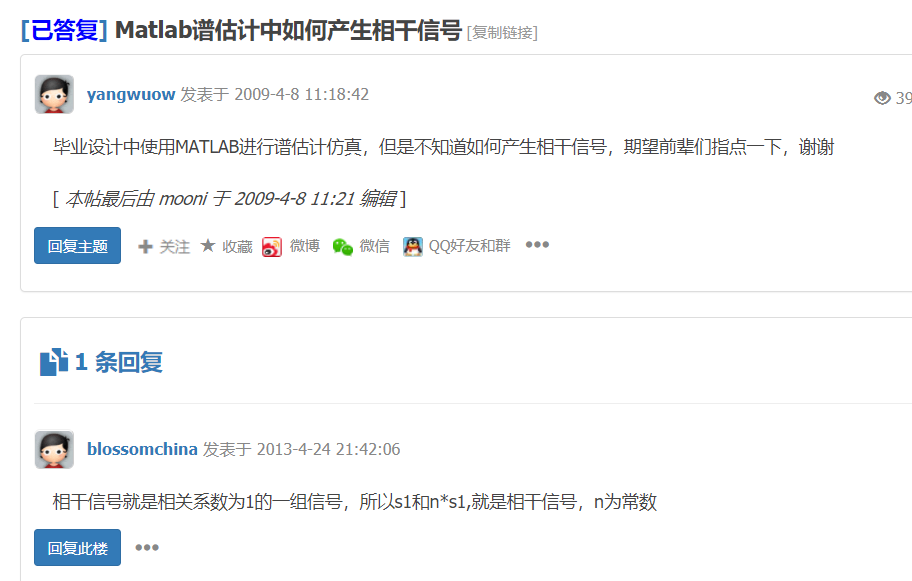2、相干信号的matlab产生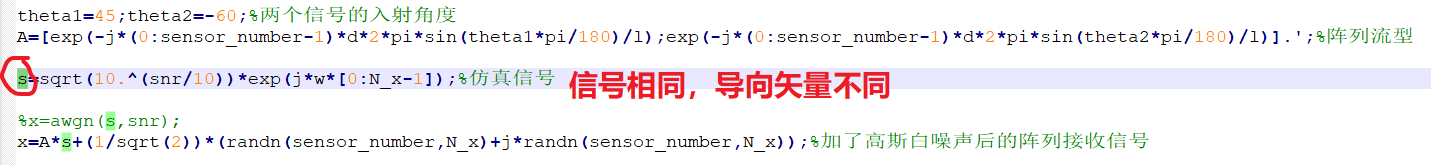展开全文•研究论文
• 均匀线阵下的相干信号的空间谱估计，采用了MUSIC算法
• 利用接收数据及其共轭信息,重新构造子阵数据矩阵,在不损失有效工作阵元情况下采用运算量小的修正C-ESPRIT方法实现非圆信号在任意初始相位下的相干信号估计。仿真结果表明,VC-ESPRIT算法有效地消除信号初始相位对相干...
• 针对现有的基于压缩感知理论的测向方法,其阵元利用率较低且对信噪比要求较高,基于压缩感知理论,提出了一种信号子空间测量模型,并将其用于独立信号与相干信号同时存在的情况,实现了任意阵列测向,有效扩展了阵列孔径并...
• 相干信号空间谱估计测向Matlab 仿真研究1. 引言由于多径传播、电磁干扰等因素的影响，相干信源存在的电磁环境是经常碰到的。当空间存在相干源时，经典的超分辨DOA 估计方法：MUSIC 算法和ESPRIT 算法，已经失去了其...
• 基于空间平滑MUSIC算法的相干信号DOA估计(1) 2.3 后向空间平滑算法 后向空间平滑更准确的说是共轭后向空间平滑，它是对后向子阵列地共轭接收数据协方差矩阵进行平滑。定义第一个共轭后向子阵列由 {M,M−1,⋯ ,M−p...matlab 算法 矩阵
• 基于特征空间 ＭＵＳＩＣ算法的相干信号波达方向空间平滑估计研究论文...